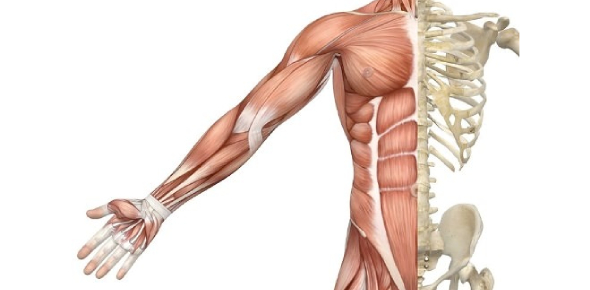# Skeletal Muscle Questions Quiz! Trivia

25 Questions | Total Attempts: 106Settings.

• 1.
Troponin and Tropomyosin wrap around which filament?
• A.

Actin

• B.

Myosin

• 2.
In the relaxed state, tropomyosin covers the binding site for actin or myosin.
• A.

Actin

• B.

Myosin

• 3.
What is myosin considered as being?
• A.

Structural

• B.

Mechanical

• C.

Enzyme

• 4.
Sarcomeres are the basic functional unit for which type of muscle?
• A.

Skeletal

• B.

Cardiac

• C.

Smooth

• 5.
The distance between what lines compose the sarcomere?
• A.

I bands

• B.

A bands

• C.

Z lines

• D.

H zones

• 6.
Innervated by alpha motor neurons and produce a force of contraction.
• A.

Extrafusal fibers

• B.

Sensors

• C.

Intrafusal fibers

• 7.
The distance from one part of a sarcomere to the same part of the next sarcomere.
• A.

A band

• B.

I band

• C.

Z line

• D.

H zone

• 8.
Regulate sensors and is innervated gamma motor neurons.
• A.

Extrafusal fibers

• B.

Sensors

• C.

Intrafusal fibers

• 9.
The entire length of the myosin filaments is known as
• A.

I band

• B.

H zone

• C.

A band

• D.

Z line

• 10.
Contractile machinery is activated by a rise in
• A.

Calcium

• B.

Magnesium

• C.

Potassium

• D.

Sodium

• 11.
What terminates the contraction?
• A.

Diffusion of calcium from cells

• B.

The coupling of calcium and sodium

• C.

Transport of calcium back into the SR

• D.

An influx of more calcium

• 12.
Produced as a result of force generated by making & breaking cross-bridges.
• A.

Passive tension

• B.

Active tension

• C.

Metabolic energy

• D.

Velocity

• 13.
What is required for active force?
• A.

Calcium pulse

• B.

Release of calcium from SR

• C.

Transport of calcium back into the SR

• D.

Extracellular fluid calcium

• 14.
Causes flaccid paralysis.
• A.

Hypercalcemia

• B.

Hypocalcemia

• 15.
Causes spasms
• A.

Hypercalcemia

• B.

Hypocalcemia

• 16.
In hypercalcemia, extracellular calcium can block Na channels preventing action potentials. This leads to flaccid paralysis.
• A.

True

• B.

False

• 17.
Is it the ATP binding that breaks cross bridges?
• A.

Yes

• B.

No

• 18.
The activity of myosin ATPase limits.
• A.

Power

• B.

Velocity

• C.

Work

• D.

Tension

• 19.
The best measurement of preload is?
• A.

Passive tension

• B.

Sarcomere length

• C.

Effort

• D.

Active tension

• 20.
Check all that apply for isotonic contraction.
• A.

No change of force

• B.

Tension does not increase

• C.

Tension does increase

• D.

Length decreases

• 21.
Fibers for endurance!
• A.

Type I

• B.

Type II

• 22.
Holding a heavy load uses less energy than repetitive drumming.
• A.

True

• B.

False

• 23.
Ways to regulate force...
• A.

• B.

• C.

Frequency

• D.

Recruitment

• 24.
This way to regulate the force is not available for the heart.
• A.

• B.

• C.

Frequency

• D.

Recruitment

• 25.
The mechanism that provides ATP for contraction.
• A.

Direct phosphorylation

• B.

Glucose

• C.

Oxidation of fatty acids

Related TopicsBack to top